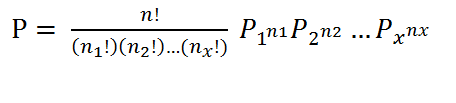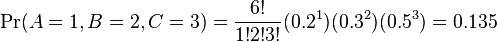# Multinomial Distribution: Definition, Examples

Share on

Probability Distributions > Multinomial Distribution

The multinomial distribution is used to find probabilities in experiments where there are more than two outcomes.

## Binomial vs. Multinomial Experiments

The first type of experiment introduced in elementary statistics is usually the binomial experiment, which has the following properties:

• Fixed number of n trials.
• Each trial is an independent event.
• Only two outcomes are possible (Success and Failure).
• Probability of success (p) for each trial is constant.
• A random variable Y= the number of successes.

A multinomial experiment is almost identical with one main difference: a binomial experiment can have two outcomes, while a multinomial experiment can have multiple outcomes.

Example: You roll a die ten times to see what number you roll. There are 6 possibilities (1, 2, 3, 4, 5, 6), so this is a multinomial experiment. If you rolled the die ten times to see how many times you roll a three, that would be a binomial experiment (3 = success, 1, 2, 4, 5, 6 = failure).

A binomial experiment will have a binomial distribution. A multinomial experiment will have a multinomial distribution.

## Multinomial Distribution Example

Three card players play a series of matches. The probability that player A will win any game is 20%, the probability that player B will win is 30%, and the probability player C will win is 50%. If they play 6 games, what is the probability that player A will win 1 game, player B will win 2 games, and player C will win 3?

Use the following formula to calculate the odds (Need help? Check out our tutoring page!):where:

• n = number of events
• n1 = number of outcomes, event 1
• n2 = number of outcomes, event 2
• n3 = number of outcomes, event x
• p1 = probability event 1 happens
• p2 = probability event 2 happens
• px = probability event x happens

Using the data from the question, we get:

• n = 12 (6 games total).
• n1 = 1 (Player A wins).
• n2 = 2 (Player B wins).
• n3 = 3 (Player C wins).
• p1 = 0.20 (probability that Player A wins).
• p2 = 0.30 (probability that Player B wins).
• p3 = 0.50 (probability that Player C wins).

Putting this into the formula, we get:Check out our YouTube channel for hundreds of statistics help videos!

## References

Beyer, W. H. CRC Standard Mathematical Tables, 28th ed. Boca Raton, FL: CRC Press, p. 532, 1987.
Papoulis, A. Probability, Random Variables, and Stochastic Processes, 2nd ed. New York: McGraw-Hill, 1984.

CITE THIS AS:
Stephanie Glen. "Multinomial Distribution: Definition, Examples" From StatisticsHowTo.com: Elementary Statistics for the rest of us! https://www.statisticshowto.com/multinomial-distribution/
---------------------------------------------------------------------------Need help with a homework or test question? With Chegg Study, you can get step-by-step solutions to your questions from an expert in the field. Your first 30 minutes with a Chegg tutor is free!